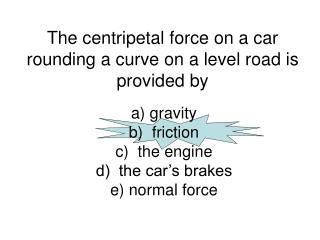DownloadDownload PresentationThe centripetal force on a car rounding a curve on a level road is provided by

# The centripetal force on a car rounding a curve on a level road is provided by

Download Presentation## The centripetal force on a car rounding a curve on a level road is provided by

- - - - - - - - - - - - - - - - - - - - - - - - - - - E N D - - - - - - - - - - - - - - - - - - - - - - - - - - -
##### Presentation Transcript

1. The centripetal force on a car rounding a curve on a level road is provided by a) gravityb) frictionc) the engined) the car’s brakese) normal force

2. On a “banked” turn, what is providing the centripetal force on a car? • friction and tension • friction and gravity • friction and normal force • friction only

3. The moon’s mass is only 1.2% of the earth’s mass. Relative to the gravitational force the earth exerts on the moon, the gravitational force the moon exerts on the earth is a) smallerb) the samec) greaterd) depends on whether the moon is made of cheese or not

4. The moon’s mass is only 1.2% of the earth’s mass. The gravitational acceleration the earth experiences compared to the gravitational acceleration the moon experiences is • smaller b) the same c) greater

5. A bucket on a water wheel with a radius of 2.5 m makes one complete revolution in 4.2 seconds. How fast is the bucket moving? a)7.48 m/s b)3.7 m/s c)2.6 m/s d)1.87 m/s v = d/t d = Circumference = 2πr v = 2π(2.5)/4.2 v = 3.7 m/s

6. A 0.5 kg ball moves in a circle 40 cm in radius at a speed of 4.0 m/s. Its centripetal acceleration is F = mv2/r ma = mv2/r a = v2/r a = 42/.4 a = 40 m/s2 a) 10 m/s2 b) 20 m/s2 c) 30 m/s2 d) 40 m/s2

7. If the mass of Sputnik was doubled, by what factor would the force change if it was to remain in the same circular orbit? • two times greater • four times greater • half as much • one-fourth as much • no change

8. Two small masses that are 10 cm apart attract each other with a force of 10 N. When they are 5 cm apart, with what force will they attract each other? (G = 6.67  10-11 Nm2/kg2) a) 5 N b) 2.5 N c) 20 N d) 40 N

9. An object moves in a circular path. If the radius is doubled while keeping the speed the same, then the centripetal force must be: a) two times moreb) half as muchc) four times mored) one-fourth as muche) the same

10. A planet of mass 3  1028 kg is in orbit around a star with mass 3  1030kg. The radius of the orbit is 1.5  1010m. The speed of the planet in orbit is a) 6.03  1048 m/sb) 1.15  1012 m/sc) 1.15  104 m/sd) 1.16  105 m/s

11. The speed of the planet from the previous question is 1.16  105 m/s. What is the period of the planet’s orbit? a)8.12  105 sb)4.21  106 sc)3.23  103 sd)2.74  103 s

12. The radius of the path of an object in constant circular motion is doubled. The centripetal force needed if its speed remains the same is a) half as great as it was beforeb) the same as beforec) twice as great as befored) four times as great as before

13. Consider a roller coaster doing a loop. What provides the centripetal force? • Friction • Normal force • Tension • Gravity

14. Consider a roller coaster at the top of a loop. What provides the centripetal force? • Friction and Gravity • Normal force and Friction • Tension and Normal Force • Gravity and Normal Force • Centripetal Force and Gravity